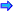# Predefined Functions

One of the objects of good programming style should be to minimize the amount of repeated coding that must done. By this is meant that the programmer should try to use functions that are already defined. The language C++ has many different functions already built into libraries. These functions are called predefined functions. To use them all that needs to be done is to attach their header file to the program with a #include. For example Standard C++ has a library that is called cmath. (In Classical C++ the header was called math.h). Some of the predefined functions in the library cmath are:cmath Library FunctionsOpen table as spreadsheet

Function

Purpose

ceil(double theValue)

Returns the smallest integer greater that theValue

cos(double theValue)

Returns the double that is the cosine of theValue

sin(double theValue)

Returns the double that is the sine of theValue

fabs(double theValue)

Returns the absolute value of theValue

floor(double theValue)

Returns the largest integer less than theValue

pow(float theValue, int thePower)

Returns theValue raised to the exponent thePower

exp(double theValue)

Returns the double that is e raised to theValue power

log(double theValue)

Returns the double that is log base e of theValue

sqrt(double theValue)

Returns the double that is the square root of theValueSuppose that a program was needed that would output the squares and cubes of all of the numbers from 1 up to a value specified by the user. This could be done by using the following calculations: theNumber * theNumber and theNumber * theNumber * theNumber to output. But using pow() from the cmath library, these expressions could be written as: pow(theNumber,2) and pow(theNumber,3). See powers.cpp.

Two other C++ built in libraries that you may find useful are: cstdlib and cctype. (These files were stdlib.h and ctype.h in Classical C++) Some examples of predefined functions in these libraries are the following:cstdlib Library FunctionsOpen table as spreadsheet

Functions

Purpose

abs(double theValue)

Returns the absolute value of theValue

rand()

Returns a number between 0 and 1Suppose that you wanted to obtain a collection of random numbers that are between two values like 1 and 25. The predefined function rand( ) from the cstdlib library could be used. However since rand( ) returns random numbers between 0 and 1, the function needs the following modification to get these values: rand() % 25 + 1. (The 1 was needed because you wanted to start at 1 and go to 25.) For example see random.cpp. Compile and run the program. Did the outcome appear as you expected? Run the program several times. Observe the first and last number each time. Were these values the same each time the program is run? This is one of the problems with rand(). To overcome this problem a call to the computer's clock could be used to generate numbers that are more random. (This process will not be discussed in these lectures.)cctype Library FunctionsOpen table as spreadsheet

Functions

Purpose

tolower(char theValue)

Returns the lower case value of theValue

toupper(char theValue)

Returns the upper case value of theValue

islower(char theValue)

Returns true when theValue is lower case and false otherwise

isupper(char theValue)

Returns true when theValue is upper case and false otherwise

isdigit(char theValue)

Returns true when theValue is a digit and false otherwiseSuppose that you wanted to ensure that the user was entering a number and not some non digit character. For this, you could use the predefined function: isdigit( ) from the library cctype. For example see checkDigit.cpp. Think how useful this could be used for checking the amount of money someone entered into your program, or their phone number or their credit card number.

In general, when you use a predefined function in a program, you do not know what its algorithms are. As a result the pseudo code of a program that contains a predefined function would not show how the function works. In general, since this function is not being coded by the programmer, it may not be listed in the structure chart just like you do not list the use of setw() from the header file iomanip.

There are additional C++ built-in libraries but they will not be discussed in this part of the lectures.